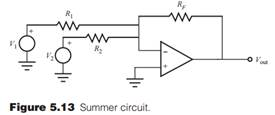Create an Account

Home / Questions / If a voltage source V3 is inserted between ground and the non inverting input in the summe...

If a voltage source V3 is inserted between ground and the non inverting input in the summer circuit shown in Figure 513 what is the resulting equation for the output voltage Vout in terms of the in

If a voltage source V3 is inserted between ground and the non-inverting input in the summer circuit shown in Figure 5.13 , what is the resulting equation for the output voltage Vout in terms of the input voltages Vand V2 if R1 = R2 = RF?Jul 27 2020 View more View LessSubscribe To Get Solution